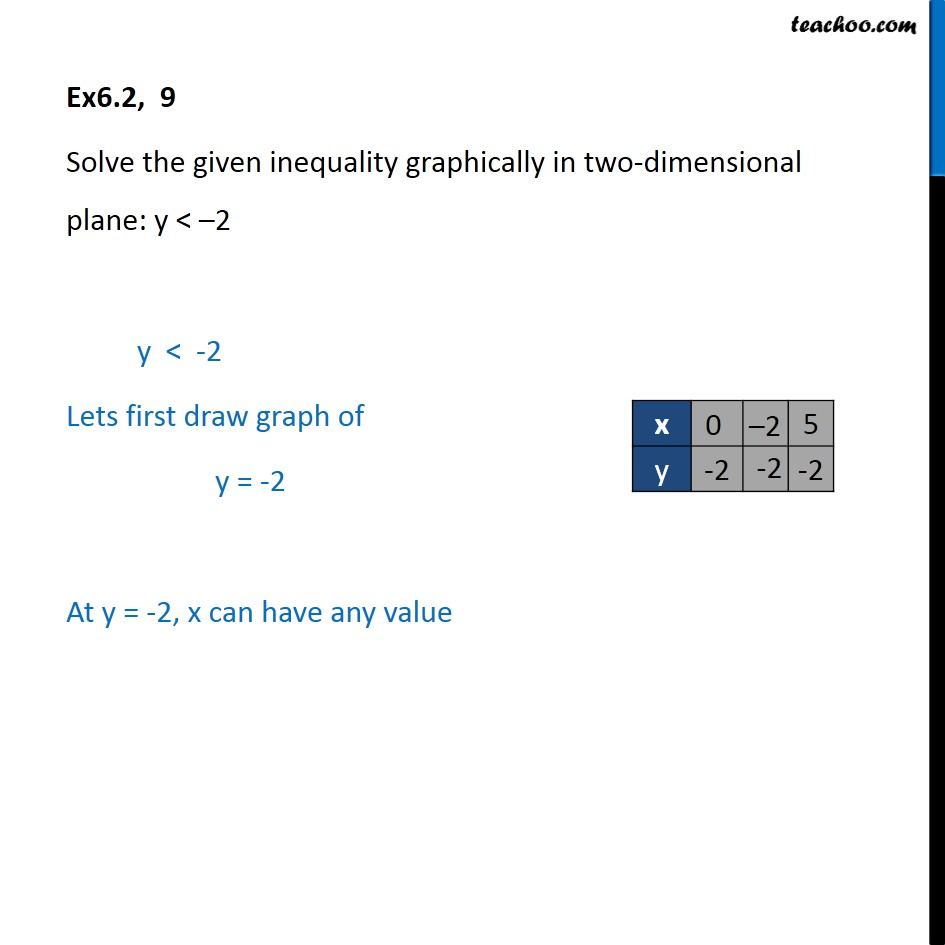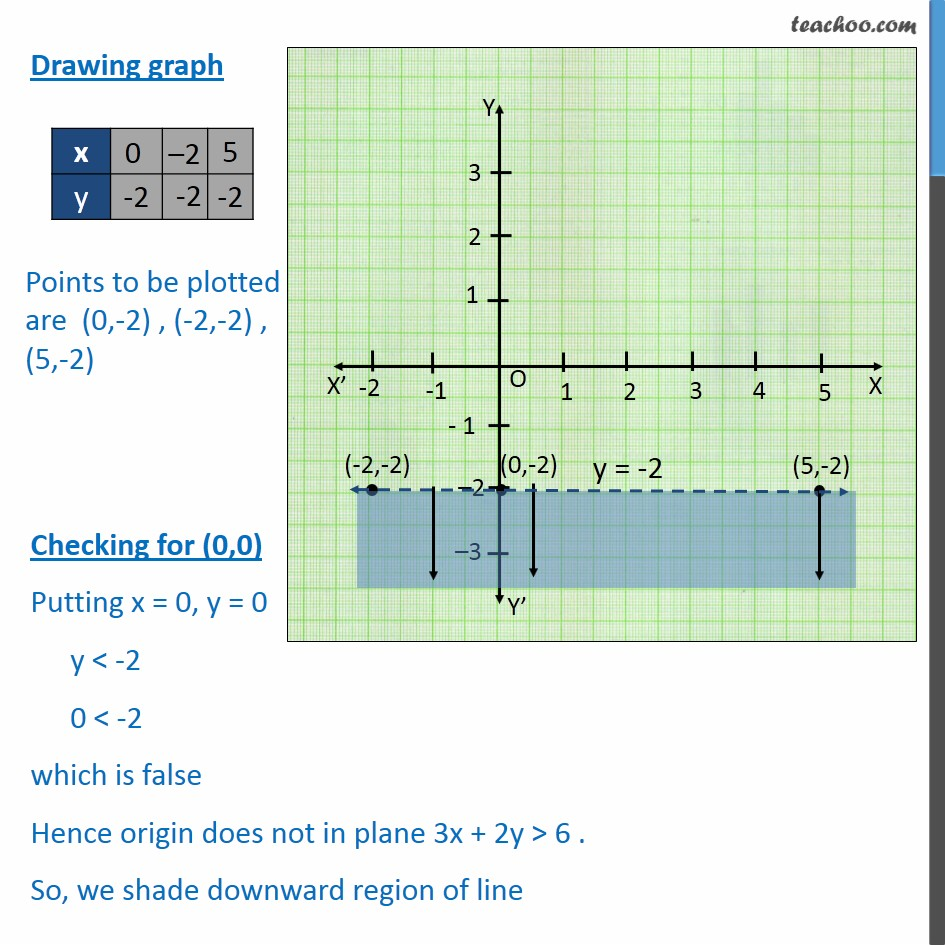Solving Linear Inequality Graphically

Chapter 5 Class 11 Linear Inequalities
Serial order wiseLearn in your speed, with individual attention - Teachoo Maths 1-on-1 Class

### Transcript

Ex6.2, 9 Solve the given inequality graphically in two-dimensional plane: y < –2 y < -2 Lets first draw graph of y = -2 At y = -2, x can have any value Drawing graph Checking for (0,0) Putting x = 0, y = 0 y < -2 0 < -2 which is false Hence origin does not in plane 3x + 2y > 6 . So, we shade downward region of line